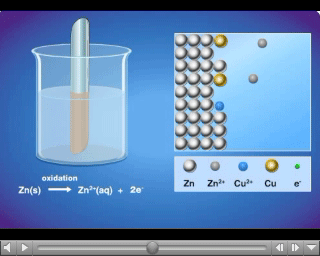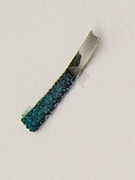# Electrochemistry

-Introduction

Step 1:

-Investigating redox reactions

-Practice with redox reactions

-Reduction tendencies of metal ions

Step 2:

-Electron transfer

-Electrochemical cell

-Practice with cells

-Powering a stopwatch

Step 3:

-Measuring potentials

-Calculating potentials

-Practice with potentials

-Applying potentials

Step 4:

-Non-standard conditions

-Practice with non-standard cells

Feedback Form:

Electrochemistry Tutorial: Galvanic Cells and the Nernst Equation >> Step 2: Explaining the electron transfer process

 < Previous | Next >

# Electrochemistry: Galvanic Cells and the Nernst Equation

## Step 2: Explaining the electron transfer process

The following video from the McGraw-Hill website shows what happens during a redox reaction at a molecular level.© McGraw-Hill. Movie opens in a separate window and requires the Flash player.

To understand how the redox reaction can be used to generate an electric current, let us consider one of the reactions we have just investigated. You have noticed that when a strip of Zn was placed into the copper solution, a black deposit of copper metal was formed on the surface of zinc. (See image below).Let’s revisit the Zn and Cu2+ reaction from the previous movie:

Under the conditions shown in the movie, i.e., by dipping Zn metal in a copper nitrate solution, no useful work can be obtained from the chemical energy involved in the reaction. The energy is released as heat.

In the next section, we’ll see how to harness this energy to do electrical work, i.e. we’ll learn how to make a galvanic cell (battery).

 < Previous | Next >